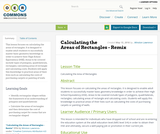Updating search results...

# 3 Results

View
Selected filters:
• Rectangular Aeas
RemixUnrestricted Use
CC BY
Rating
0.0 stars

This lesson focuses on using the properties of rectangles to calculate area. It is designed to aid adult students to successfully master and apply basic geometry knowledge in a professional setting (construction and related) and can also contribute to achieving High School Equivalency (HSE).
Areas to be covered include properties of rectangles and squares; definition of ‘square’; right-angled triangles; calculating areas of rectangles and right-angled triangles; decomposition of area into manageable units; and calculating costs. Students will apply this knowledge to calculating the costs of purchasing building materials.

Subject:
Mathematics
Material Type:
Interactive
Lesson Plan
Author:
david cowell
08/08/2019
RemixUnrestricted Use
CC BY
Rating
0.0 stars

This lesson focuses on calculating the areas of rectangles. It is designed to enable adult students to successfully master basic geometry knowledge in order to achieve their High School Equivalency (HSE). Areas to be covered include types of polygons, quadrilaterals, rectangles; calculating areas of rectangle and calculating costs. Students will apply this knowledge to practical areas of their lives such as calculating the costs of purchasing carpets or painting of walls

Subject:
Mathematics
Material Type:
Interactive
Lesson Plan
Author:
Winston Lawrence
08/08/2019Unrestricted Use
CC BY
Rating
0.0 stars

This lesson focuses on calculating the areas of rectangles. It is designed to enable adult students to successfully master basic geometry knowledge in order to achieve their High School Equivalency (HSE). Areas to be covered include types of polygons, quadrilaterals, rectangles; calculating areas of rectangle and calculating costs. Students will apply this knowledge to practical areas of their lives such as calculating the costs of purchasing carpets or painting of walls

Subject:
Mathematics
Material Type:
Interactive
Lesson Plan
Author:
Winston Lawrence